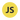In this article, we will learn everything about JavaScript Regular Expressions which will summarize the essential concepts and utilize them off with examples.

We will cover: ⁣

### 1. What is RegExp or Regular expression?

A regular expression also `regex` or `regexp` for short, is a string of characters that allows you to create patterns of string that help match, locate, and manage text in a very performant way.⁣

By using a regular expression we can:

• search a text from a string.
• replace a substrings from a string.
• extract an information from a string. ⁣ One common use of regex is an email address and form validation.⁣

### 2. Two ways to create regex patterns⁣

We can create RegExp or Regular expression using two way:

#### 2.1 Enclosing the expression between two `//`.

``let regex = /ab+c/;``

#### 2.2 Calling the constructor function of `RegExp` object.

``let regex = new RegExp(ab + c);``

### 3. Modifiers

Modifiers are used to perform case-insensetive and global searches.

#### 3.1 `g` -> Perform a global match. It finds all matches rather than stopping after first match.

``````const str = "Is this all there is?";
const pattern = /is/g;
console.log(str.match(pattern));``````

Using `g` in regExp will match the case-sensitive character search for "is" in a string.

``Output: ["is", "is"]``

#### 3.2 `i` -> Performs case -insensitive matching.

``````const str = "Is this all there is?";
const pattern = /is/gi;
console.log(str.match(pattern));``````

Using `i` in regExp will match the case-insensitive character search for "is" in a string.

``````Output: ["Is", "is", "is"]
``````

#### 3.3 `m` -> Perform multiline matching.

``````const str = "\nIs th\nis it?";
const pattern = /is/m;
console.log(str.match(pattern));``````

`m` does a multiline search for "is" at the beginning of each line in a given string.

``Output: ["is"]``

### 4. Brackets

Brackets in `regexp` are used to find a range of characters within a string.

#### 4.1 [abc]: Find any character between the bracket.

``````const str = "Is this all there is?";
const pattern = /[h]/g;
console.log(str.match(pattern));
//["h", "h"]``````

#### 4.2 `[^abc]`: Find any NOT characters between the bracket.

``````const str = "Is this all there is?";
const pattern = /[^this]/g;
console.log(str.match(pattern));
//["I", " ", " ", "a", "l", "l", " ", "e", "r", "e", " ", "?"]``````

#### 4.3 [0-9]: Find any character between the bracket(any digits).

``````const str = "Is this all there is? There is 0 also.";
const pattern = //g;
console.log(str.match(pattern));
//["0"]``````

#### 4.4 `[^0-9]`: Find any NOT characters between the bracket(any non-digits).

``````const str = "Is this all there is? There is 0 also.";
const pattern = /[^0]/g;
console.log(str.match(pattern));
//["I", "s", " ", "t", "h", "i", "s", " ", "a", "l", "l", " ", "t", "h", "e", "r", "e", " ", "i", "s", "?", " ", "T", "h", "e", "r", "e", " ", "i", "s", " ", " ", "a", "l", "s", "o", "."]``````

#### 4.5 (x|y): Find any of the alternate specified

``````const str = "Is this all there is? There is 0 also.";
const pattern = /[0|this]/g;
console.log(str.match(pattern));
//["s", "t", "h", "i", "s", "t", "h", "i", "s", "h", "i", "s", "0", "s"]``````

### 5. Metacharacters

Metacharacters are character with special meanings

• `.`: Metacharacters finds a single character, except newline or line terminator.
• `\w`: Finds a word character.
• `\W`: Finds a non-word character.
• `\d`: Finds a digit character.
• `\D`: Finds a non-digit character.
• `\s`: Finds a whitespace character.
• `\S`: Finds a non-whitespace character.
• `\b`: Finds a match at the beginning/end of a word.
• `\B`: Finds a match but not at the beginning/end of a word.
• `\0`: Finds a `NULL` character.
• `\n`: Finds a new line character.
• `\f`: Finds a form feed character.
• `\r`: Finds a carriage return character.
• `\t`: Finds a tab character.
• `\v`: Finds a vertical tab charater.
• `\xxx`: Finds the charater specified by an `octal` number `xxx`.
• `\xdd`: Finds the character specified by a `hexadecimal` number `dd`.
• `\udddd`: Finds the `Unicode` character specified by a hexadecimal number `dddd`.

### 6. Quantifiers

Quantifiers specify how many instances of a character, group, or character class must be present in the input for a match to be found.

• `n+`: Matches any string that contains atleast one n.
• `n*`: Matches any string that contains zero or more occurance of `n`.
• `n?`: Matches any string that contains zero or one occurance of `n`.
• `n{X}`: Matches any string that contains a sequence of `X` `n's`.
• `n{X,Y}`: Matches any string that contains a sequence of `X` to `Y` `n's`.
• `n{X,}`: Matches any string that contains a sequence of atleast `X` `n's`.
• `n\$`: Matches any string with `n` at the end of it.
• `?=n`: Matches any string that is followed by a specific string `n`.
• `?!n`: Matches any string that is not followed by a specific string `n`.

### 7. RegExp Common Examples

Here are few commpon examples of RegExp:

#### 7.1 Extracting numbers from string:

``````const input = "252number27anothernumber";
const output = input.match(/\d+/g);
console.log("Output", output);
//Output ["252", "27"]``````

#### 7.2 Matching alpha numeric string:

``````const input = "alphaNumberic123";
const output = input.match(/^[a-z0-9]+\$/i);
console.log("Output", output);
//Output: ["alphaNumberic123"]

var a = "Test123*** TEST";
var b = a.replace(/[^a-z0-9]/gi, "");
console.log(b);
//Output: Test123TEST``````

#### 7.3 Validating Email address:

``````const re = /\S+@\S+\.\S+/;
console.log(re.test("iam@pratapsharma.io"));
//Output: true

const re = /\S+@\S+\.\S+/;
console.log(re.test("iam@pratapsharma.io"));
//Output: false``````

#### 7.4 Expression to match a URL?

``````const expression = /[-a-zA-Z0-9@:%._~#=]{1,256}\.[a-zA-Z0-9()]{1,6}\b([-a-zA-Z0-9()@:%_.~#?&//=]*)?/gi;
const url = "www.pratapsharma.io";

console.log(expression.test(url));
//Output: true

const expression = /[-a-zA-Z0-9@:%._~#=]{1,256}\.[a-zA-Z0-9()]{1,6}\b([-a-zA-Z0-9()@:%_.~#?&//=]*)?/gi;
const url = "pratapsharma";

console.log(expression.test(url));
//Output: false``````

### Conclusion

I hope this study kick-started your Regex selection within your JavaScript projects, getting the benefits that Regex offers for formatting and validating strings.

You may also like my other articles:

If you liked the article, feel free to share it to help others find it!